# Integer Complexity

The complexity of a natural number n is the minimum number of ones required to write an arithmetic expression for n using ones, addition, multiplication and parentheses. It is denoted by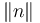$\left\| n \right\|$. The integer complexity has a corresponding sequence in The On-Line Encyclopedia of Integer Sequences: A005245. Jānis Iraids has calculated the sequence up to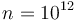$n=10^{12}$ and the data is available in an interactive form.

Equivalently,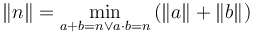$\left\| n \right\| = \min_{a+b = n \vee a\cdot b = n} {(\left\| a \right\| + \left\| b \right\|)}$.

## Logarithmic complexity

Since the integer complexity of n is bounded by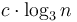$c\cdot\log_3{n}$, the logarithmic complexity$\left\| n \right\|_{log} = \frac{\left\| n \right\|}{\log_3 {n}}$ is of interest as a sort of efficiency measure of n.

## Distribution of logarithmic complexity

### The "best" numbers

The numbers on the left side of the logarithmic complexity distribution curves are simple and their expressions are known. These numbers are also known as sequence A000792 on The On-Line Encyclopedia of Integer Sequences.

### The "worst" numbers

The numbers represented on the right side of the logarithmic complexity distribution curves are more complex. The number represented by the rightmost nonzero column is the smallest number of the given complexity. These numbers are also known as sequence A005520 on The On-Line Encyclopedia of Integer Sequences.

It has been conjectured that starting from the 26th all these numbers are primes.

## Some numerical data

This table summarizes what is known about the "best" and "worst" numbers as well as the distribution of numbers of equal complexity.$n$ A005520(n)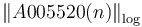$\left\|A005520(n)\right\|_{\log}$ PrimeQ[A005520(n)]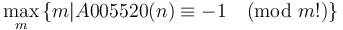$\underset{m}{\operatorname{max}} \, \{m | A005520(n) \equiv -1 \pmod{m!}\}$ A000792(n) A133374(n) A005421(n)
1 1 - False 2 1 0 1
2 2 3.17 True 0 2 0 1
3 3 3.00 True 2 3 0 1
4 4 3.17 False 0 4 0 1
5 5 3.41 True 3 6 1 2
6 7 3.39 True 2 9 2 3
7 10 3.34 False 0 12 2 2
8 11 3.67 True 3 18 7 6
9 17 3.49 True 3 27 10 6
10 22 3.55 False 0 36 14 7
11 23 3.85 True 4 54 31 14
12 41 3.55 True 3 81 40 16
13 47 3.71 True 4 108 61 20
14 59 3.77 True 3 162 103 34
15 89 3.67 True 3 243 154 42
16 107 3.76 True 3 324 217 56
17 167 3.65 True 4 486 319 84
18 179 3.81 True 3 729 550 108
19 263 3.75 True 4 972 709 152
20 347 3.76 True 3 1458 1111 214
21 467 3.75 True 3 2187 1720 295
22 683 3.70 True 3 2916 2233 398
23 719 3.84 True 6 4374 3655 569
24 1223 3.71 True 4 6561 5338 763
25 1438 3.78 False 0 8748 7310 1094
26 1439 3.93 True 6 13122 11683 1475
27 2879 3.72 True 6 19683 16804 2058
28 3767 3.74 True 4 26244 22477 2878
29 4283 3.81 True 3 39366 35083 3929
30 6299 3.77 True 3 59049 52750 5493
31 10079 3.69 True 7 78732 68653 7669
32 11807 3.75 True 4 118098 106291 10501
33 15287 3.76 True 4 177147 161860 14707
34 21599 3.74 True 6 236196 214597 20476
35 33599 3.69 True 5 354294 320695 28226
36 45197 3.69 True 3 531441 486244 39287
37 56039 3.72 True 5 708588 652549 54817
38 81647 3.69 True 4 1062882 981235 75619
39 98999 3.72 True 5 1594323 1495324 105584
40 163259 3.66 True 3 2125764 1962505 146910
41 203999 3.68 True 5 3188646 2984647 203294
42 241883 3.72 True 3 4782969 4541086 283764
43 371447 3.68 True 4 6377292 6005845 394437
44 540539 3.66 True 3 9565938 9025399 547485
45 590399 3.72 True 6 14348907 13758508 763821
46 907199 3.68 True 7 19131876 18224677 1061367
47 1081079 3.72 True 5 28697814 27616735 1476067
48 1851119 3.65 True 6 43046721 41195602 2057708
49 2041199 3.71 True 7 57395628 55354429 2861449
50 3243239 3.66 True 5 86093442 82850203 3982054
51 3840479 3.70 True 7 129140163 125299684 5552628
52 6562079 3.64 True 7 172186884 165624805 7721319
53 8206559 3.66 True 6 258280326 250073767 10758388
54 11696759 3.65 True 5 387420489 375723730 14994291
55 14648759 3.66 True 5 516560652 501911893 20866891
56 22312799 3.64 True 6 774840978 752528179 29079672
57 27494879 3.66 True 5 1162261467 1134766588 40534895
58 41746319 3.63 True 7 1549681956 1507935637 56439467
59 52252199 3.65 True 5 2324522934 2272270735 78684930
60 78331679 3.63 True 7 3486784401 3408452722 109675955
61 108606959 3.62 True 7 4649045868 4540438909 152788554
62 142990559 3.63 True 6 6973568802 6830578243 213072724
63 203098319 3.62 True 6 10460353203 10257254884 297002458
64 273985919 3.62 True 6 13947137604 13673151685 413944635
65 382021919 3.61 True 7 20920706406 20538684487 577354385
66 495437039 3.62 True 7 31381059609 30885622570 804919055
67 681327359 3.62 True 8 41841412812 41160085453 1122274894
68 1006290359 3.60 True 5 62762119218 61755828859 1565492145
69 1406394359 3.60 True 5 94143178827 92736784468 2182968270
70 1857794399 3.60 True 7 125524238436 123666444037 3044509482
71 2728424159 3.59 True 7 188286357654 185557933495 4247469161
72 3743197919 3.59 True 7 282429536481 278686338562 5923889964
73 5008227839 3.59 True 8 376572715308 371564487469 8263996299
74 6872690159 3.59 True 7 564859072962 557986382803 11530495182
75 9839491199 3.58 True 9 847288609443 837449118244 16084845369
76 13485479039 3.58 True 6 1129718145924 1116232666885 -
77 16724776319 3.59 True 9 1694577218886 1677852442567 -
78 24679458719 3.58 True 7 2541865828329 2517186369610 -
79 35524698479 3.57 True 6 3389154437772 3353629739293 -
80 44211625919 3.59 True 7 5083731656658 5039520030739 -
81 62391692159 3.58 True 8 7625597484987 7563205792828 -
82 93753213119 3.57 True 7 10167463313316 10073710100197 -
83 121551917759 3.57 True 7 15251194969974 15129643052215 -
84 163539961199 3.57 True 7 22876792454961 22713252493762 -
85 250585241759 3.56 True 7 30502389939948 30251804698189 -
86 320429329919 3.57 True 8 45753584909922 45433155580003 -
87 424847520719 3.57 True 7 68630377364883 68205529844164 -
88 630371064959 3.56 True 8 91507169819844 90876798754885 -
89 872573642639 3.56 True 7 137260754729766 136388181087127 -

## Concerning the best expressions

There have been conjectures as to what can be the best expression of a number. Notably, it was conjectured, that for prime numbers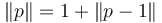$\left\|p\right\| = 1+\left\|p-1\right\|$

and$\left\|2p\right\| = \min{\{2+\left\|p\right\|, 1+\left\|2p-1\right\|\}}$.

Even though the intuition behind the hypotheses is very natural - if the number is written as a sum, one of the addends should be very small, i.e. 1, since "expected contribution" to the other addend (as an addend to one of its multipliers) is so much more greater - both of these hypotheses have been shown to be false. However, the smallest offending numbers are quite large: 353942783 and 10278600694, respectively.

Some more counterexamples are listed in this table:$n$ A189124(n) PrimeQ[A189124(n)]
1 353942783 True
2 516743639 False
3 1163385647 True
4 1542243239 False
5 1932319583 True
6 2336924879 True
7 3113713259 False
8 3444631199 False
9 3878989487 False
10 4103787551 False
11 4166809919 True
12 4937621453 True
13 5123340683 True
14 5170931639 False
15 5184740299 True
16 5200683263 False
17 5390865059 True
18 5455982879 True
19 5467766947 True
20 5570566315 False
21 5876676427 False
22 6020880739 False
23 6213081067 False
24 6432033887 True
25 6459553799 True
26 6545574839 True
27 6714582263 True
28 6888368878 False
29 6988649399 True
30 7349349419 False
31 7354261907 False
32 7378517519 True
33 7515851039 True
34 7657182539 True
35 7756383347 True
36 8219266919 True
37 8265240899 False
38 8267366687 False
39 8312800997 True
40 8319180029 False
41 9299744395 False
42 9307738439 False
43 9312441947 True
44 9417418919 True
45 9649914763 False
46 9687112847 True
47 9796592617 False
48 9797579279 True
49 9810679247 True
50 9816022007 False
51 9819417887 True
52 10009162939 False
53 10251400499 True
54 10278600694 False
55 10752114599 False
56 10795928723 True
57 10858961203 False
58 10870190159 False
59 10900918439 False
60 10948216919 False
61 10948217573 False
62 10982945399 True
63 11030276879 True
64 11430539819 True
65 11520231839 False
66 11879689919 True
67 12279593759 True
68 12402781733 True
69 12440572891 True
70 12464418523 False
71 12483890999 True
72 12506394959 False
73 12571726823 True
74 12580039259 True
75 12686036183 True

Up to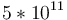$5*10^{11}$ there are 283 counterexamples (all in File:2pCounter.txt) to the second conjecture. The first 50 are:$n$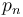$p_n$
1 10278600694
2 15497722798
3 17048096134
4 20726927638
5 21760403254
6 25899777502
7 31329368062
8 32062787998
9 32801694118
10 32869094542
11 33096145534
12 45557779858
13 46507340158
14 46539226654
15 49550857198
16 84744886894
17 88995291622
18 93431048254
19 93497874046
20 99509671438
21 111750707734
22 113050749334
23 114773208694
24 116657631358
25 116873841598
26 122110222174
27 139615908478
28 140017166494
29 141054542998
30 141121365118
31 144638031598
32 144655590778
33 145797393958
34 149190546634
35 149286208174
36 159497138734
37 161540046106
38 170485334638
39 170536762078
40 171785274334
41 171786551542
42 186119672822
43 186603284734
44 188076442354
45 198433351294
46 209236359742
47 220007539582
48 223183537198
49 223671709726
50 225394226782

## Programs

A program to calculate complexity in base {1,+,-,*} is found here: File:Minus.txt.